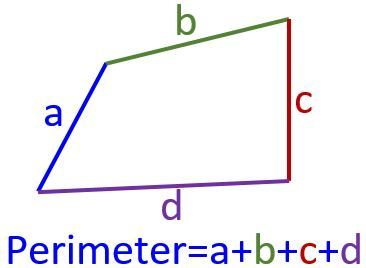# Perimeter

PERIMETER    It is the distance around a figure. To find the perimeter add up the lengths of all sides.

Keywords: fencing, framing, edge, border.### Perimeter of a Polygon### CF17      Composite Figures Area and Perimeter 17The sum of the interior angles of a quadrilateral is 360 degrees.Find Missing Dimensions of Rectangles

(a)Use the area formula of a rectangle to find its length.

Find the length of the rectangle shown.Area 20 cm².

A = L x W

Replace A with 20 and W with 4

20 = 4 L

divide both sides by 4

So, the length L is 5 inches.

(b)Use the perimeter formula for a rectangle to find its length.

Find the length of the rectangle shown.P = 2(L+W)

Replace P with 18 and W with 4

18 = 2(L+4)

divide both sides by 2

9 = (L+4)

So, the length L is 5 inches.

GE02          Perimeter and area of a square 1

### SQ13 Find the perimeter of a square given its area

GE03          Perimeter and area of a square 2

GE01          Perimeter and area of a square 3

AR38          Perimeter and area of a rectangle 1

AR39          Perimeter and area of a rectangle 2

AR40          Perimeter and area of a rectangle 3

### AR09 Finding width of a rectangle from perimeter and length

GE07          Length and width of a rectangle given area

### GE08Length and width of a rectangle given perimeter

AR36          Find the missing dimension in each rectangle 2

AR37          Find the missing dimension in each rectangle 3

AR33          Area and perimeter word problems

AR35          MINIQUIZ Find the missing dimension in each rectangle.

AR34          Perimeter and area of a rectangle WP

GE05          Perimeter and area of a rectangle 1

GE06          Perimeter and area of a rectangle 2

GE04          Perimeter and area of a rectangle with decimals

GE10          Area of a Rhombus 1

GE11          Area of a Rhombus 2

XXXX         Area of a parallelogram 1

GE15          Area of a trapezoid 1

GE14          Area of a trapezoid 2

GE13          Area of a trapezoid with decimals

XXXX         Find the missing dimension of a trapezoid

GE12          Area of a Kite

GE18          Area of a triangle 1

GE17          Area of a Triangle 2

GE16          Area  of a triangle with decimalsMs. Garcia Book, Practice Exercises:

PERIMETER:P1,  P2,  P3,  P4,  P5,  P6,  P7,  P8,  P9,  P10,  P11,  P12,  P13,  P14,  P15,  P16,  P17,  P18,  P19,  P20,  P21,  P22.